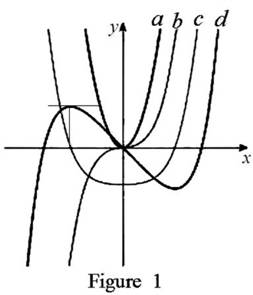# The curves f , f ′ , f ′ ′ and f ‴ on given graph and give proper explanation.### Single Variable Calculus: Concepts...

4th Edition
James Stewart
Publisher: Cengage Learning
ISBN: 9781337687805### Single Variable Calculus: Concepts...

4th Edition
James Stewart
Publisher: Cengage Learning
ISBN: 9781337687805

#### Solutions

Chapter 2.7, Problem 42E
To determine

## To Identify: The curves f,f′,f′′ and f‴ on given graph and give proper explanation.

Expert Solution

In the given graph,d=f, c=f, b=f and a=f.

### Explanation of Solution

Graph:

The given graph is shown as in Figure 1,Observation:

Observe the graph of c and d carefully.

The point where c(x)=0 is the same point where graph of d(x) has horizontal tangent.

Recall that the derivative of a function is zero where the function has a horizontal tangent.

c(x) is the derivative of the graph d(x).

Thus, d(x)=c(x). (1)

Observe the graph of b and c carefully.

The slope of c has negative value when x<0. Only the curve b has negative value when x<0. Also, it is observed that the slope of c has positive value when x>0 while the curve b has positive value when x>0.

Thus, c(x)=b(x). (2)

Observe the graph of b and a carefully.

The slope of b has positive value when x{0}. Only the curve a has positive value when x.

Thus, b(x)=a(x). (3)

From (1), (2) and (3), it is concluded that, d(x)=a(x),d(x)=b(x) and d(x)=c(x)

Thus, d=f, c=f, b=f and a=f.

### Have a homework question?

Subscribe to bartleby learn! Ask subject matter experts 30 homework questions each month. Plus, you’ll have access to millions of step-by-step textbook answers!